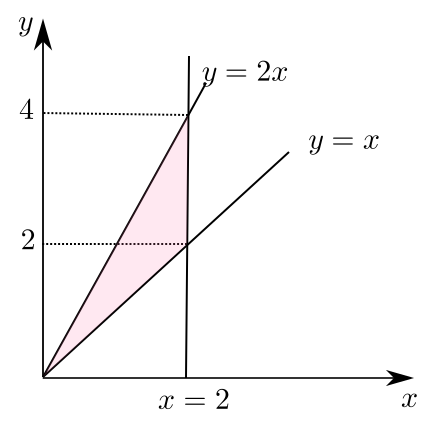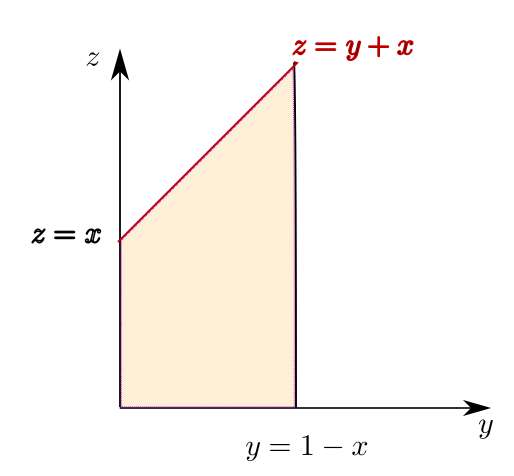# 猴子也能听懂的三重积分换序问题

## 从二重积分说起

【例子】改变积分次序：

$$\int_0^2\mathrm{d}x\int_x^{2x}f(x,y)\mathrm{d}y$$

### 如何画出二重积分的积分区域$$D_1:0<y<2\ \and \ \frac y2 <x<y$$

$$D_2:2<y<4\ \and \ \frac y2 <x<2$$

$$I_{D_1}+I_{D_2} = \int_{0}^{2} \mathrm{d} y \int_{\frac{1}{2} y}^{y} f(x, y) \mathrm{d} x+\int_{2}^{4} \mathrm{d} y \int_{\frac{1}{2} y}^{2} f(x, y) \mathrm{d} x$$

## 三重积分

【例子】（从里到外）将 $z,y,x$ 顺序换为 $y,x,z$ 顺序：

$$\int_{0}^{1} \mathrm{d} x \int_{0}^{1-x} \mathrm{d} y \int_{0}^{x+y} f(x, y, z) \mathrm{d} z$$

$$y=0\to y=1-x\\z=0\to z=x+y$$D_1:\left\{{\begin{aligned} 0 \leq y \leq 1-x\\ 0 \leq z \leq x \end{aligned}}\right.\ D_2:\left\{\begin{array}{l} z-x \leq y \leq 1-x \\ x \leq z \leq 1 \end{array}\right.

$$\int_{0}^{1} \mathrm{d} x\left(\int_{0}^{x} \mathrm{d} z \int_{0}^{1-x} f(x, y, z) \mathrm{d} y+\int_{x}^{1} \mathrm{d} z \int_{x-x}^{1-x} f(x, y, z) \mathrm{d} y\right)$$

$$\int_{0}^{1} \mathrm{d} z \int_{z}^{1} \mathrm{d} x \int_{0}^{1-x} f(x, y, z) \mathrm{d} y+\int_{0}^{1} \mathrm{d} z \int_{0}^{x} \mathrm{d} x \int_{z-x}^{1-x} f(x, y, z) \mathrm{d} y$$